Courses
Courses for Kids
Free study material
Free LIVE classes
More

# NCERT Solutions for Class 10 Science Chapter 3## NCERT Solutions for Class 10 Science Chapter 3 - Metals and Non-metals

Here are all the Class 10 Science Chapter 3 NCERT Solutions. This solution includes questions, answers, photos, examples of the complete Chapter 3, Metals and Non-Metals of Science taught in Class 10. Chapter 3 Metals and Non-Metals is an important chapter that students should learn in class 10th. These solutions are also prepared in accordance with the guidelines of the NCERT textbook. For you to revise the entire syllabus and score a higher score, all the exercise questions given in NCERT Solutions for Class 10 Science chapter 3 are there for you available in Vedantu’s website. You can download Class 10 Maths NCERT Solutions to help you to revise complete syllabus and score more marks in your examinations.

## Important Topics Covered in Class 10 Science Chapter 3

• Physical and Chemical Properties of Metals and Non-metals

• How Do Metals and Non-metals React?

• Occurrence of Metals

• Corrosion

Last updated date: 24th May 2023
Total views: 416.7k
Views today: 5.76k
Watch videos on
NCERT Solutions for Class 10 Science Chapter 3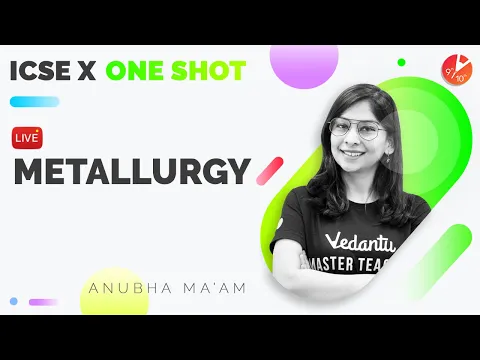Metallurgy Full Chapter in One Shot - ICSE Class 10 Chemistry/Science Chap 7 | Semester 2 | Vedantu
Vedantu 9&10
SubscribeShare
4.4K likes
144.7K Views
1 year ago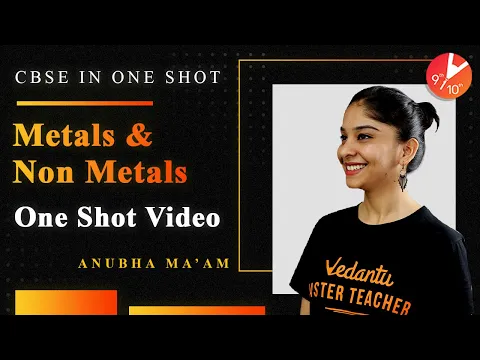Metals and Non Metals in One Shot | CBSE Class 10 Chemistry | Science Chapter 3 NCERT | Vedantu
Vedantu 9&10
7.3K likes
227.5K Views
2 years ago
Play Quiz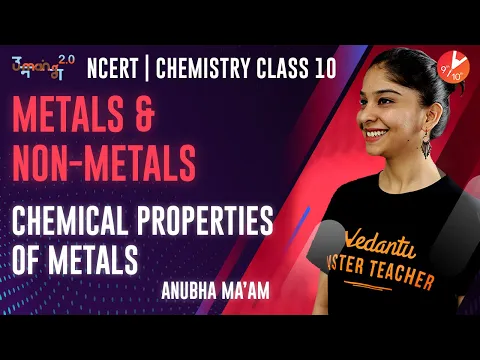Metal and Non Metals L2 | Chemical Properties of Metals | CBSE Class 10 Chemistry | Umang Vedantu
Vedantu 9&10
5K likes
157.2K Views
2 years ago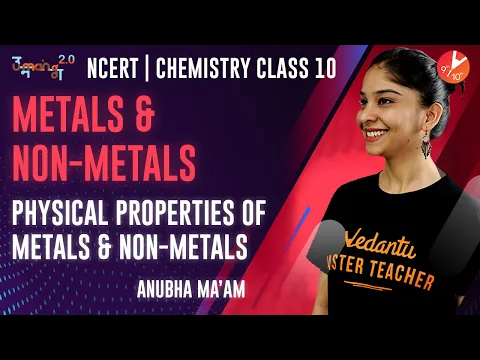Metal and Non Metals L1 | Physical Properties of Metals and Non Metals | CBSE Class 10 Chemistry
Vedantu 9&10
7.3K likes
218.3K Views
2 years ago
See More## NCERT Solutions for Class 10 Science Chapter 3 - Metals and Non-metals

Intext Questions:

1. Give an example of a metal which

i. is a liquid at room temperature.

Ans: Mercury

ii. can be easily cut with a knife.

Ans: Potassium

iii. is the best conductor of heat.

Ans: Copper and Silver

iv. is a poor conductor of heat.

Ans: Mercury.

2. Explain the meanings of malleable and ductile.

Ans: Malleable: Property of a metal due to which it can be beaten into thin sheets is known as malleability and the metal is known to be malleable.

Ductile: Property of a metal due to which it can be beaten into thin pipes/wire is known as ductility and the metal is known to be ductile.

Intext Questions:

1. Why sodium is kept immersed in kerosene oil?

Ans: Sodium is a very reactive metal. It reacts with the air and catches fire as soon as it comes in contact with it. Hence, to avoid such accidents sodium is kept immersed in kerosene oil.

2. Write equations for the reactions of

i. iron with steam

Ans: $3 \mathrm{Fe}(\mathrm{s})+4 \mathrm{H} 2 \mathrm{O}(\mathrm{g}) \rightarrow \mathrm{Fe3O4}(\mathrm{s})+4 \mathrm{H} 2(\mathrm{~g})$

ii. calcium and potassium with water

Ans: $\mathrm{Ca}(\mathrm{s})+2 \mathrm{H}_{2} \mathrm{O}(\mathrm{l}) \rightarrow \mathrm{Ca}(\mathrm{OH})_{2}(\mathrm{aq})+\mathrm{H}_{2}(\mathrm{~g})$

$2 \mathrm{~K}(\mathrm{~s})+2 \mathrm{H}_{2} \mathrm{O}(\mathrm{l}) \rightarrow 2 \mathrm{KOH}(\mathrm{aq})+\mathrm{H}_{2}(\mathrm{~g})+$ heat

3. Samples of four metals A, B, C and D were taken and added to the following solution one by one. The results obtained have been tabulated as follows:

 Metals Iron (II) Sulphate Copper (II) Sulphate Zinc Sulphate Silver Nitrate A No Reaction Displacement B Displacement No Reaction C No Reaction No Reaction No Reaction D No Reaction No Reaction No Reaction No Reaction

Use the Table above to answer the following questions about metals A, B, C and D.

According to the activity series the most reactive metal displaces the least reactive metal. according to the given table we can say that:

$\mathrm{A}$ is less reactive than iron but more reactive with copper.

$\mathrm{A}+\mathrm{FeSO}_{4} \rightarrow \text { No reaction }$

$\mathrm{A}+\mathrm{CuSO}_{4} \rightarrow \text { Single Displacement }$

B is more reactive than iron but less reactive than zinc.

$\mathrm{B}+\mathrm{FeSO}_{4} \rightarrow \text { Single Displacement }$

$\mathrm{B}+\mathrm{ZnSO}_{4} \rightarrow \text { No reaction }$

$\mathrm{C}$ is less reactive than iron, copper and zinc but more reactive with silver

$\mathrm{C}+\mathrm{FeSO}_{4} \rightarrow \text { No reaction }$

$\mathrm{C}+\mathrm{CuSO}_{4} \rightarrow \text { No reaction }$

$\mathrm{C}+\mathrm{ZnSO}_{4} \rightarrow \text { No reaction }$

$\mathrm{C}+\mathrm{AgNO}_{3} \rightarrow \text { Single Displacement }$

D is less reactive than iron, copper,zinc, and silver

$\mathrm{D}+\mathrm{FeSO}_{4} \rightarrow \text { No reaction }$

$\mathrm{D}+\mathrm{CuSO}_{4} \rightarrow \text { No reaction }$

$\mathrm{D}+\mathrm{ZnSO}_{4} \rightarrow \text { No reaction }$

$\mathrm{D}+\mathrm{AgNO}_{3} \rightarrow \text { No Reaction }$

i. Which is the most reactive metal?

Ans: B is the most reactive metal.

${\text{B}} + {\text{FeS}}{{\text{O}}_4} \to {\text{Single Displacement}}$

ii. What would you observe if B is added to a solution of copper (II) sulphate?

Ans: When B is added to a solution of copper (II) sulphate then it shows the single displacement because B is more reactive than iron.

${\text{B}} + {\text{CuS}}{{\text{O}}_4} \to {\text{Single Displacement}}$

iii. Arrange the metals A, B, C and D in the order of decreasing reactivity.

Ans: ${\text{B}} > {\text{A}} > {\text{C}} > {\text{D}}$

4. Which gas is produced when dilute hydrochloric acid is added to a reactive metal? Write the chemical reaction when iron reacts with dilute $\mathrm{H}_{2} \mathbf{SO}_{4}$.

Ans: Iron is more reactive than hydrogen according to the reactivity series. So, when iron comes in contact with dilute $\mathrm{H}_{2} \mathrm{SO}_{4}$ it replaces hydrogen to form iron sulphate and in the reaction it releases hydrogen gas.

${\text{F}}{{\text{e}}_{(s)}} + {{\text{H}}_2}{\text{S}}{{\text{O}}_{4(aq)}} \to {\text{FeS}}{{\text{O}}_{4(aq)}} + {H_{2(g)}}$

5. What would you observe when zinc is added to a solution of iron (II) sulphate? Write the chemical reaction that takes place.

Ans: According to the reactivity series we know zinc is more reactive than iron. So, when zinc comes in contact with iron sulphate solution it replaces iron and forms zinc sulphate. And the colour of solutions turns brown from green.

${\text{Z}}{{\text{n}}_{(s)}} + {\text{FeS}}{{\text{O}}_{4(aq)}} \to {\text{ZnS}}{{\text{O}}_{4(aq)}} + F{e_{(s)}}$

Intext Exercise:

i. Write the electron-dot structures for sodium, oxygen and magnesium.

Ans: Sodium, oxygen and magnesium atomic numbers are 11, 8 and 12. The electron dot structures are below:

Sodium (2,8,1) = $\overset{\text{X}}{\mathop{\text{Na}}}\,$

Oxygen (2,6) =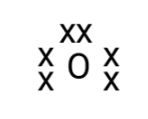Magnesium (2,8,2) = $\overset{\text{XX}}{\mathop{\text{Mg}}}\,$

ii. Show the formation of ${\text{N}}{{\text{a}}_{\text{2}}}{\text{O}}$and MgO by the transfer of electrons.

Ans: Both of the compounds will form an ionic bond.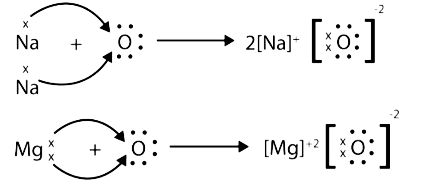iii. What are the ions present in these compounds?

Ans: In the $\mathrm{Na}_{2} \mathrm{O} \text {, the ions } 2 \mathrm{Na}^{+} \text {and } \mathrm{O}^{2} \text { - and in } \mathrm{MgO} \text { are } \mathrm{Mg}^{2+} \text { and } \mathrm{O}^{2} \text { - }$.

2. Why do ionic compounds have high melting points?

Ans: Ionic compounds have high electrostatic force between the molecules due to which they are very closely packed and rigid. This tight packing results in ionic compounds having high melting points.

Refer to page 53.

1. Define the following terms.

i. Minerals:

Ans: The minerals which are present as a mixture of metallic compounds and extractable impurities are known as ores and the compound of a metal found in nature is called mineral.

ii. Ore:

Ans: The minerals from which the metals can be conveniently and economically extracted are known as ores.

iii. Gangue:

Ans: The ore contains some impurities like sand, clay and rocky material. These impurities are called gangue or matrix. Gangue is removed before the metallurgical process starts.

2. Name two metals which are found in nature in the free state.

Ans: Silver and Gold are found in free state as their reactivity potential is very low.

For example: Silver(Ag), Gold (Au), and Platinum(Pt).

3. What chemical process is used for obtaining a metal from its oxide?

Ans: More reactive metals are reduced by the electrolysis process. moderate reactive metals can be reduced by either carbon or carbon monoxide. Metal oxides are reduced by using suitable reducing agents such as carbon or by highly reactive metals to displace the metals from their oxides. For example, zinc oxide is reduced to metallic zinc by heating with carbon.

$\mathrm{ZnO}_{(s)}+C_{(s)} \stackrel{\Delta}{\longrightarrow} \mathrm{Zn}_{(s)}+\mathrm{CO}_{(g)}$ or

$\mathrm{ZnO}+\mathrm{CO} \rightarrow \mathrm{Zn}+\mathrm{CO}_{2}$

Thermite process: Chromium oxides are reduced to chromium by treating it with aluminium powder. In this case, aluminium displaces manganese from its oxide.

${\text{C}}{{\text{r}}_2}{{\text{O}}_3} + 2{\text{Al}} \to {\text{A}}{{\text{l}}_2}{{\text{O}}_3} + 2{\text{Cr}} + {\text{heat}}$

Refer to page 55.

1. Metallic oxides of zinc, magnesium and copper were heated with the following metals.

 Metals Zinc Magnesium Copper Zinc Oxide - - - Magnesium Oxide - - - Copper Oxide - - -

In which cases will you find displacement reactions taking place?

Ans:

 Metals Zinc Magnesium Copper Zinc Oxide No reaction Displacement No reaction Magnesium Oxide No reaction No reaction No reaction Copper Oxide Displacement Displacement No reaction

2. Which metals do not corrode easily?

Ans: Metals with less reactivity such as silver, platinum and gold.

3. What are alloys?

Ans: An alloy is a homogenous mixture of two metals or metal and non-metals. They are formed by melting the metals and mixing them and then solidifying them into alloy.

Example: Bronze is an alloy of copper and tin.

Exercise Questions

1. Which of the following pairs will give displacement reactions?

(a) $\mathbf{NaCl}$ solution and copper metal

(b) $\mathbf{MgC}{{\mathbf{l}}_{\mathbf{2}}}$ solution and aluminium metal

(c) $\mathbf{FeS}{{\mathbf{O}}_{\mathbf{4}}}$ solution and silver metal

(d) $\mathbf{AgN}{{\mathbf{O}}_{\mathbf{3}}}$ solution and copper metal.

Ans: (d) $\mathbf{AgN}{{\mathbf{O}}_{\mathbf{3}}}$ solution and copper

2. Which of the following methods is suitable for preventing an iron frying pan from rusting?

(a) Applying grease

(b) Applying paint

(c) Applying a coating of zinc

(d) all of the above.

Ans: (c) Applying a coating of zinc

3. An element reacts with oxygen to give a compound with a high melting point. This compound is also soluble in water. The element is likely to be

(a) calcium

(b) carbon

(c) silicon

(d) iron

Ans: (a) calcium

4. Food cans are coated with tin and not with zinc because

(a) zinc is costlier than tin.

(b) zinc has a higher melting point than tin.

(c) zinc is more reactive than tin.

(d) zinc is less reactive than tin.

Ans: (c) zinc is more reactive than tin.

5. You are given a hammer, battery, bulb, wires and a switch.

(a) How could you use them to distinguish between samples of metals and non-metals?

Ans: Metals are naturally malleable. We can beat the given sample with the help of a hammer. If it turns into thin sheets, it is metal; otherwise, it is nonmetal.

Metals carry electricity. when we use a battery, a lamp, wires, and a switch to create a circuit. If the bulb glows, it indicates that the sample is metallic due to liberated electrons. If the bulb does not light up, this indicates that the sample is non-metallic.

(b) Assess the usefulness of these tests in distinguishing between metals and nonmetals.

Ans: The physical properties of metals and non-metals are represented by these tests. We can easily distinguish between them based on their physical characteristics.

6. What are amphoteric oxides? Give two examples of amphoteric oxides.

Ans: Oxides which can react with both acid and base is known as amphoteric oxide.

Example: Zinc oxide $(ZnO)$ and Aluminum oxide$\left(\mathrm{Al}_{2} \mathrm{O}_{3}\right)$

7. Name two metals which will displace hydrogen from dilute acids, and two metals which will not.

Ans: More reactive metals will displace hydrogen from its dilute acids. for example : Iron and Zinc.

$\mathrm{Fe}+2 \mathrm{HCl} \rightarrow\mathrm{FeCl}_{2}+\mathrm{H}_{2}$

$\mathrm{Zn}+2 \mathrm{HCl} \rightarrow\mathrm{ZnCl}_{2}+\mathrm{H}_{2}$

Least reactive metals will not displace hydrogen from its dilute acids. for example : copper and silver

$\mathrm{Cu}+\mathrm{HCl} \rightarrow$ No Reaction

$\mathrm{Ag}+\mathrm{HCl} \rightarrow$ No Reaction

8. In the electrolytic refining of a metal M, what would you take as the anode, the cathode and the electrolyte?

Ans: In the electrolytic refining of a metal M:

i. Cathode → Pure metal M

ii. Anode → Impure metal M

ii. Electrolyte → Salt Solution of the metal M

9. Pratyush took sulphur powder on a spatula and heated it. He collected the gas evolved by inverting a test tube over it, as shown in figure below.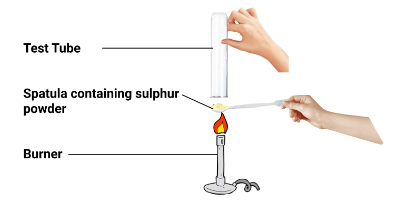(a) What will be the action of gas on

i. dry litmus paper?

Ans: no reaction with dry litmus paper.

ii. moist litmus paper?

Ans: Moist blue litmus will turn red due to the formation of sulphur dioxide. Sulphur dioxide is acidic oxide when it will react with water converts into sulphurous acid.

(b) Write a balanced chemical equation for the reaction taking place.

Ans: $\mathrm{S}_{(s)}+\mathrm{O}_{2(g)} \longrightarrow \mathrm{SO}_{2(g)}$

$\mathrm{SO}_{2(g)}+\mathrm{H}_{2} \mathrm{O}_{(l)} \longrightarrow \underset{\text { Sulphur dioxide }}{\longrightarrow} \mathrm{H}_{2} \mathrm{SO}_{3(a q)}$

10. State two ways to prevent the rusting of iron.

Ans: The process of degrading metals in presence of air and water is known as rusting. Two ways in which rusting can be prevented are as follows:

1. Painting: It prevents iron from coming in direct contact with air and water.

2. Galvanization: In this process iron is coated with a layer of zinc.

11. What type of oxides is formed when nonmetals combine with oxygen?

Ans: Non-metals combine with oxygen and form acidic oxides which when combined with water turns into acidic solution.

12. Give reasons

(a) Platinum, gold and silver are used to make jewelers.

Ans: These metals are very less reactive and are lustrous which makes them suitable for making jewelry.

(b) Sodium, potassium and lithium are stored under oil.

Ans: They are highly reactive metals and catch fire as soon as it comes in contact with the air. Hence, to avoid any accidents these metals are kept under oil.

(c) Aluminium is a highly reactive metal, yet it is used to make utensils for cooking.

Ans: Aluminium is highly reactive and they react with oxygen present in atmosphere to form a stable compound $\mathrm{Al}_{2} \mathrm{O}_{3}$. Along with this, they are very good conductor of heat. Therefore, aluminium is used in making utensils for cooking.

(d) Carbonate and sulphide ores are usually converted into oxides during the process of extraction.

Ans: Extracting metals from their oxides are easier as compared to their carbonate and sulphides. Therefore, ores are usually converted into oxides by the process of roasting and calcination.

13. You must have seen tarnished copper vessels being cleaned with lemon or tamarind juice. Explain why these sour substances are effective in cleaning the vessels.

Ans: Copper forms basic copper carbonate ${\text{(CuC}}{{\text{O}}_{\text{3}}}{\text{.Cu(OH}}{{\text{)}}_{\text{2}}}{\text{)}}$ when it combines with damp carbon dioxide in the air. It turns green, and the copper vase loses its gleaming brown finish. The presence of citric acid in the lemon or tamarind neutralizes the basic copper carbonate and dissolves the layer.

14. Differentiate between metals and non-metals.

Ans: Differences between metals and non-metals are as follows:

 METALS NON-METALS Metals are electropositive. Non-metals are electronegative. They react with water to form oxides and hydroxides. Some metals react with cold water, some with hot water, and some with steam. $2{\text{Na}} + 2{{\text{H}}_2}{\text{O}} \to 2{\text{NaOH}} + {{\text{H}}_2} \uparrow$ They do not react with water. They react with oxygen to form basic oxides$4{\text{Na}} + {{\text{O}}_2} \to 2{\text{N}}{{\text{a}}_2}{\text{O}}$ese have ionic bonds. They react with oxygen to form acidic or neutral oxides.${\text{C}} + {{\text{O}}_2} \to {\text{C}}{{\text{O}}_2}$These have covalent bonds They react with dilute acids to form a salt and evolve hydrogen gas. However, Cu, Ag, Au, Pt, Hg do not react.$2{\text{Na}} + 2{\text{HCl}} \to 2{\text{NaCl}} + {{\text{H}}_2} \uparrow$. They do not react with dilute acids. These are not capable of replacing hydrogen. They react with the salt solution of metals. Depending on their reactivity, displacement reaction can occur${\text{CuS}}{{\text{O}}_4} + {\text{Zn}} \to {\text{ZnS}}{{\text{O}}_4} + {\text{Cu}}$. These react with the salt solution of non-metals They act as reducing agents.${\text{Na}} \to {\text{N}}{{\text{a}}^ + } + {{\text{e}}^ - }$ These act as oxidizing agents.${\text{C}}{{\text{l}}_2} + 2{{\text{e}}^ - } \to 2{\text{C}}{{\text{l}}^ - }$

15. A man went door to door posing as a goldsmith. He promised to bring back the glitter of old and dull gold ornaments. An unsuspecting lady gave a set of gold bangles to him which he dipped in a particular solution. The bangles sparkled like new but their weight was reduced drastically. The lady was upset but after a futile argument the man beat a hasty retreat. Can you play the detective to find out the nature of the solution he had used?

Ans: The man is using Aqua Regia which is 3 parts of $\mathrm{HCL}$ and 1 part of $\mathrm{HNO}_{3}$ solution. Gold is the least reactive metal but when we put it in aqua regia the upper most layer gets dissolved in the solution and the inner layer comes out due to which it weighs less than before but is glitterier.

16. Give reasons why copper is used to make hot water tanks and not steel (an alloy of iron).

Ans: In the reactivity reaction copper comes below hydrogen and hence, it will not react with the water present/stored. On the other hand, steel which is an alloy of iron will react with the steam and can get corroded easily.

Important Points Covered in This Chapter

• Metals and non-metals are two types of elements. Metals are shiny, malleable, ductile, and excellent heat and electricity conductors. Except for mercury, which is a liquid at normal temperature, all are solids.

• By losing electrons to nonmetals, metals can generate positive ions.

• Basic oxides are formed when metals react with oxygen. Aluminum oxide and zinc oxide exhibit both basic and acidic oxide characteristics. Amphoteric oxides are the name for these oxides.

• With water and dilute acids, various metals have varied reactivities.

• An activity series is a list of common metals grouped in decreasing order of reactivity.

• Metals in the Activity class above hydrogen can displace hydrogen from dilute acids.

• When non-metals receive electrons from metals, they produce negatively charged ions, which are then converted into acidic or neutral oxides.

• Dilute acids do not have hydrogen displaced by non-metals. They create hydrides when they react with hydrogen.

Benefits of NCERT for Class 10

1. These NCERT Solutions for Class 10 Science Chapter 3 – Metals and Non-metals provide accurate and easy-to-understand information.

2. These solutions contain answers to all of the practice problems found at the conclusion of NCERT Class 10 Science textbook Chapter 3 Metals and Non-metals.

3. The answers to the questions that were asked in between chapters have also been shared.

4. Vedantu's topic specialists exclusively deliver NCERT Solutions for Class 10 Science Chapter 3 – Metals and Non-metals after significant study.

5. These solutions will be beneficial for a variety of competitive exams, including JEE, NEET, and others.

## NCERT Solutions for Class 10 Science Chapter 3 - Metals and Non-metals

Solutions to the problems will help create a solid foundation for students to learn how to use metals and non-metals in their everyday lives. They will also be able to categorize the elements into metals and non-metals in relation to the properties and uses of the elements. This often involves physical properties across the two specific metal and non-metal heads.

Chapter 3 of Class 10 Science, Metals, and Non-Metals will help students understand the metal's occurrence. The extraction of ores, the extraction of metals, the extraction of minerals in the activity series, and the extraction of metals to the top of the activity series can also explain how metals can be extracted and help produce ores. Practicing various types of questions will ensure that you understand the topic after studying Chapter 3 Metals and Nonmetals. Students can download free PDFs of NCERT Solutions for both online and offline use. Download solutions for NCERT now.

You can Find the Solutions of All the Science Chapters below.

## Benefits of NCERT Solutions for Class 10 Science

Expert and professional Vedantu teachers work diligently to provide you with high-quality study materials. All solutions are designed in a well-structured manner to make the preparation of your board exam easier.

• The solutions provided by Vedantu are a vital part of all the study material for students in Class 12.

• It serves as an excellent guide to finding the best NCERT solutions.

• All solutions are of high quality and standard.

• Expert teachers are responsible for all NCERT Solutions

• All solutions are prepared in compliance with current CBSE guidelines.

• All solutions are accurate and systematic so that it is not difficult for students to learn.

## How Will Vedantu Study Materials Benefit Students?

Vedantu's study material has been designed to answer each student's questions. There are a lot of easy, moderate, and challenging problems. That's why it benefits all students. NCERT Solutions for Class 10 Science Chapter 3 study material includes the common questions and the questions asked in the previous year. This lets the student determine the distribution of questions.

## Extra Questions of Class 10 Science Chapter 3 for Practice

1. What is the difference between calcination and roasting?

2. Why are ionic compounds hard crystalline solid?

3. How can rusting of iron be prevented?

4. Why do silver metals become black after some time when exposed to air?

5. Define gangue.

6. Define alloy.

7. Define metallurgy

8. How do both metals and non-metals react?

9. What are the different steps involved in extracting pure metals from ores?

10. What are the properties of ionic compounds?

This was the complete discussion on NCERT Solutions For Class 10 Science Chapter 3- Metals And Non-Metals. We have also provided a few extra questions for students to practice. It is highly recommended to practice the questions given in the pdf and extra questions to gain a deeper understanding of the chapter.

Keep learning with Vedantu and score excellent marks in your board exams.

## FAQs on NCERT Solutions for Class 10 Science Chapter 3

1. I want to download NCERT solutions of different chapters. How can I do that?

Students can use any of the solutions by downloading the Vedantu app from the Play Store. It is available for download free of price. You can install the Vedantu app to access the NCERT solutions whenever and wherever they want to. Our experienced and competent teachers have created these solutions with the greatest focus to make your study easier for exams.

2. What are we going to study in chapter 3 of Science class 10?

Here is the list of topics and subtopics under NCERT solutions for class 10 science chapter 3 Notes for Metals and Non-Metals:

1. Physical Properties of Metals And Non-Metals

2. Chemical Properties Of Metals

3. The process of metal burning in the air

4. What happens when metals react with water?

5. What happens when metals react with acids?

6. How different metals react with one another

7. The Reactivity Series

8. How do Metals and Non-Metals react?

9. Properties of Ionic Compounds

10. Occurrence of metals

1. Extraction of metals

2. Enrichment of Ores

3. Refining of Metals

11. Corrosion

1. Prevention of Corrosion

3. List out the differences between metals and nonmetals based on their physical properties in Chapter 3 of NCERT Solutions for Class 10 Science.

Following are the differences between Metals and Non -metals based on their physical properties as discussed in Class 10 Science Chapter 3;

• Metals are malleable and can be formed into thin sheets while non-metals can’t be formed into sheets.

• Metals are ductile and can be drawn into thin wires while non-metals can’t be.

• Metals conduct heat and electricity while non-metals don't conduct them.

• Some metals are sonorous as they produce a ringing sound when struck on a hard surface while non-metals are non- sonorous.

4. Mention the uses of metals and non-metals explained in Chapter 3 of NCERT Solutions for Class 10 Science.

Uses of metals:

• Due to the property of malleability they can be formed into thin sheets.

• They can be drawn into thin wires due to ductility.

• Used for making vessels as they are good conductors of heat.

• Used for making wires as they are good conductors of electricity.

Uses of non-metals:

• Non-metals are used as insulators on wires as they don't conduct electricity.

• They are used to make storage containers for acidic substances as they don’t react with acids.

5. What is electrolytic refining?

Electrolytic refining is a method of refining impure metals using electrolysis. In this process, the impure metal which is to be refined is made as the anode and the pure metal is made the cathode. The metal salt is used in the solution to act as an electrolyte. When current is passed through the electrolyte, pure metal from the anode dissolves in the solution and an equivalent amount of pure metal from the electrolyte solution deposits on the cathode. To study more and practise questions on this topic students can download the NCERT Solution of this chapter free of cost from the vedantu website (vedantu.com).

6. How can corrosion be prevented?

Oxidation of  iron is called corrosion which  can be prevented by:

• Galvanisation- Coating steel or iron with a layer of zinc.

• Painting- Painting the metal surface makes a layer that prevents contact with air.

• OIling- OIling also prevents corrosion.

• Alloying-  A homogenous mixture of more than one type of metal, or a metal and with a nonmetal is known as an alloy. They show less conductivity when compared to pure metals. It prevents rusting of metal

7. How are metals extracted from their ores?

The metals seen in nature are extracted from their ores. Ores however are contaminated with a lot of impurities called gangue. The extraction processes depend on the differences between the physical and chemical properties of the gangue and the ores. Different metals have different reactivities. Based on this the extraction processes are applied. Very less reactive metals can be refined by heating alone. Medium reactive metals are refined by roasting and calcination. Very high reactive metals are refined by electrolytic reduction. For more details, visit Vedantu app.